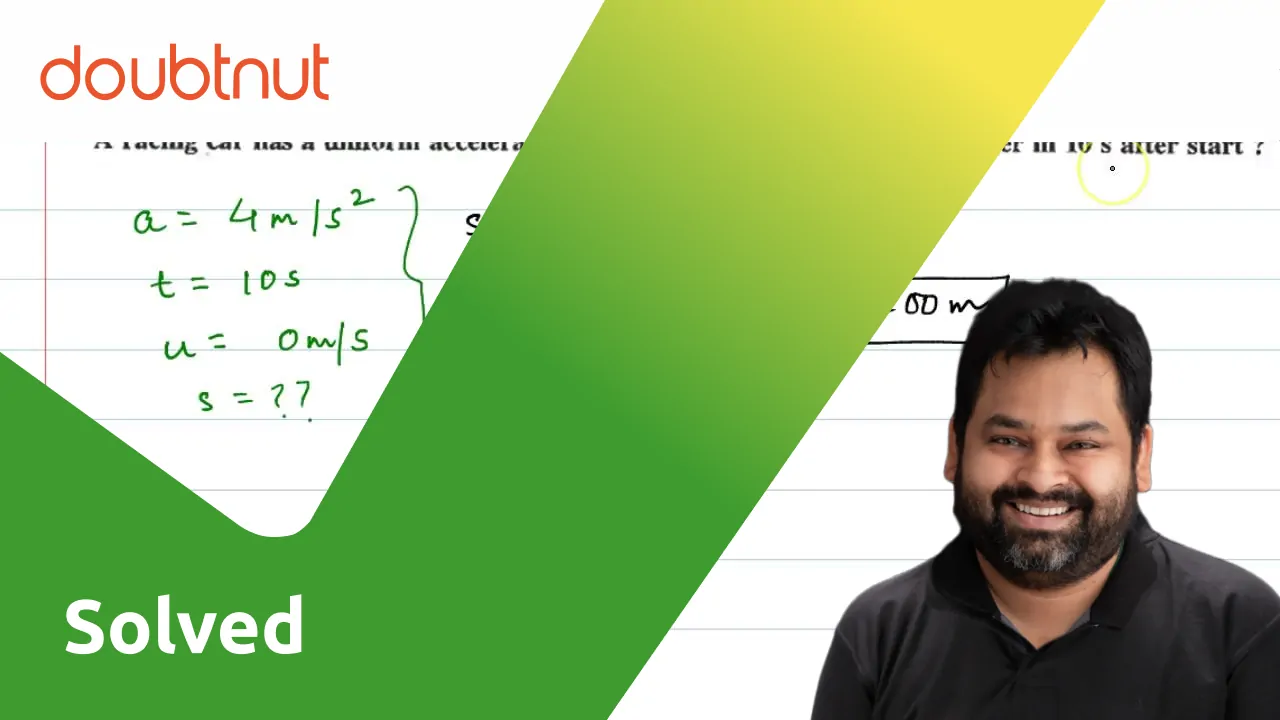Doubtnut App - Get 50 Lakh+ video solutions better than GoogleInstall Now

# A racing car has a uniform acceleration of 4m/s2. What distance will it cover in 10s after start ?

A

200 m

B

500 m

C

900 m

D

400 m

Text Solution
Verified by Experts

## Here, acceleration, a=4ms−2, time taken, t=10s initial velocity, u=0, distance, s=? From s=ut+12at2, s=0×10+12×4(10)2=200m